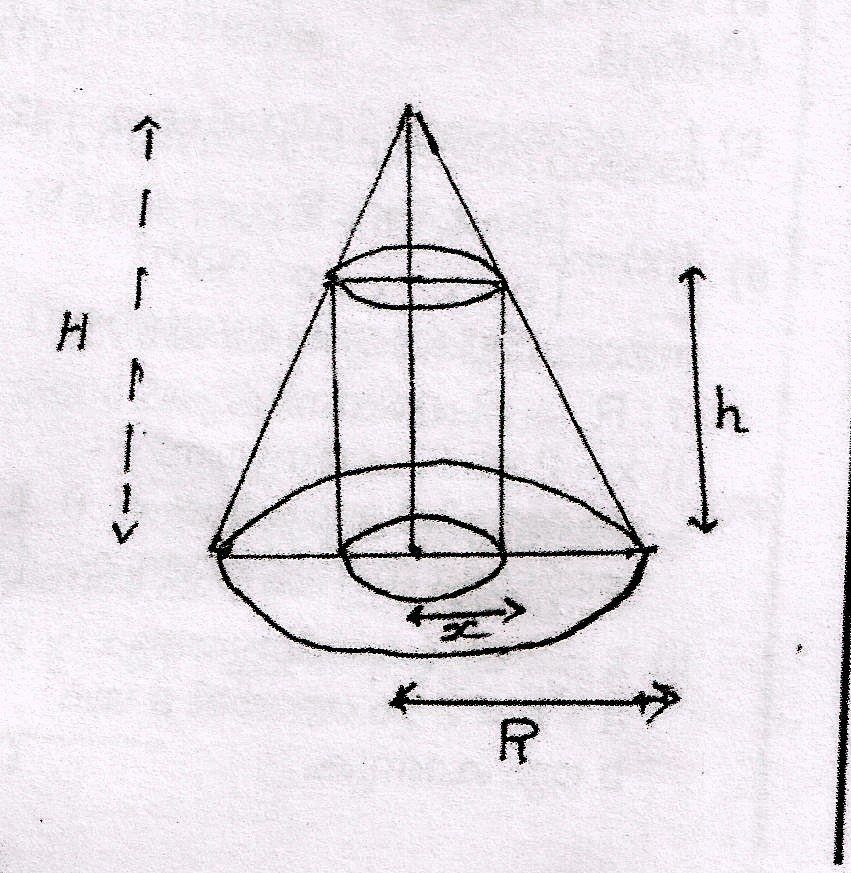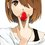# cylinder....... helpA circular cylinder is inscribed in a given cone of radius R cm and height H cm as shown in the figure Find the curved surface area S of the circular cylinder as a function of x Find the relation connecting x and R when S is maximumNote by Sonali Sukesh
7 years, 5 months ago

This discussion board is a place to discuss our Daily Challenges and the math and science related to those challenges. Explanations are more than just a solution — they should explain the steps and thinking strategies that you used to obtain the solution. Comments should further the discussion of math and science.

When posting on Brilliant:

• Use the emojis to react to an explanation, whether you're congratulating a job well done , or just really confused .
• Ask specific questions about the challenge or the steps in somebody's explanation. Well-posed questions can add a lot to the discussion, but posting "I don't understand!" doesn't help anyone.
• Try to contribute something new to the discussion, whether it is an extension, generalization or other idea related to the challenge.

MarkdownAppears as
*italics* or _italics_ italics
**bold** or __bold__ bold
- bulleted- list
• bulleted
• list
1. numbered2. list
1. numbered
2. list
Note: you must add a full line of space before and after lists for them to show up correctly
paragraph 1paragraph 2

paragraph 1

paragraph 2

[example link](https://brilliant.org)example link
> This is a quote
This is a quote
    # I indented these lines
# 4 spaces, and now they show
# up as a code block.

print "hello world"
# I indented these lines
# 4 spaces, and now they show
# up as a code block.

print "hello world"
MathAppears as
Remember to wrap math in $$ ... $$ or $ ... $ to ensure proper formatting.
2 \times 3 $2 \times 3$
2^{34} $2^{34}$
a_{i-1} $a_{i-1}$
\frac{2}{3} $\frac{2}{3}$
\sqrt{2} $\sqrt{2}$
\sum_{i=1}^3 $\sum_{i=1}^3$
\sin \theta $\sin \theta$
\boxed{123} $\boxed{123}$

Sort by:

By Similarity of Triangles,
$\displaystyle \frac{h}{R-x} = \frac{H}{R}$

$\displaystyle\Rightarrow h = \frac{H}{R} (R-x)$

Now, it is clear that,
$\displaystyle S = 2\pi x\times h$

Substitute the value of $\displaystyle h$ and get $\displaystyle S$ as a function of $\displaystyle x$.

Differentiate the function that you just derived wrt $\displaystyle x$, and put it equal to $\displaystyle 0$.

You will find a relation between $\displaystyle x$ and $\displaystyle R$.

- 7 years, 5 months ago

Right.

- 7 years, 5 months ago

2pixh = S, x = R(1-h/H)..............................................................(when S is maximum)

- 7 years, 5 months ago

S = 2pix ; x= R[1-h/H]

- 7 years, 5 months ago

need help fast

- 7 years, 5 months ago

put H/R =k k=(H-h)/x from this h=H-kx after this S=2pixh put h=H-kx differentiate with respect to x and put dS/dx=0 from this x=R/2

- 7 years, 5 months ago

thanks 2 all

- 7 years, 5 months ago

right 2pixh = S, x = R(1-h/H)..........(when S is maximum)

- 7 years, 5 months ago